### Site swap mathematics laboratory

Daniel Kahneman in Thinking, Fast and Slownoted that the remembering mind rates experiences using a peak-end rule. Although I had not known this particular fact when I designed laboratory 15 five years ago, I had always shared George M. Cohan's belief that one should "always leave them laughing when you say goodbye."Jackie juggles three in cascade pattern

A class that ends on a pleasant activity makes for better memories looking back on the course. SC 130 Physical Science Laboratory 15 is both fun and yet is also a chance to introduce an mathematical model that is very different.Beverleen throws one high

Laboratory fifteen in physical science sought to push the boundaries on the mathematical box for the students. In laboratory one a quote from Freeman Dyson was used to start a journey through the mathematical models that explain physical systems. Dyson calculated how an electron ought to behave. Later someone went into a laboratory and the electron behaved as predicted by the mathematical model.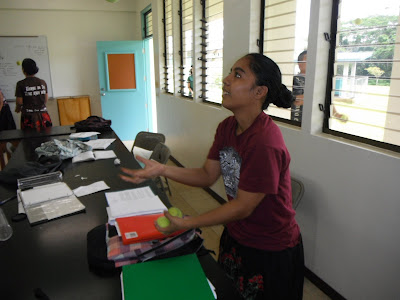Jackie multiplexes, something I had seen only two other students do before, the most recent in December 2010. Two balls were caught in her left hand, one ball is high, she then throws the two simultaneously, returning to a cascade pattern.

In laboratory two a linear model predicted the location of a rolling ball. In laboratory three a falling ball obeyed a quadratic mathematical relationship. The behavior of a marble rolling off of a banana leaf obeyed a square root relationship. And in laboratory four the marbles knew what to do in order to mathematically conserve momentum. Sound, the relative depth of an image, and Ohm's law all exhibited linear relationships.Gasma

There are other mathematical relationships that govern physical systems. There are systems that are modeled by exponential and logarithmic functions. The path of a RipStik formed a sine wave on a sheet of paper. There are exotic functions such as the hyperbolic sine and hyperbolic cosine. Some systems are best described by complex variables that include a real and an imaginary component. Many of these systems are beyond the mathematical scope of this course.Ketruth

The relationships described above are algebraic mathematical models. Much of the mathematics curriculum is centered on algebra in part because algebra is important to describing the physical world. There are, however, other mathematical models, non-algebraic models. This laboratory seeks to broaden the students mathematical horizons by introducing a mathematical model and notation that is not algebraic. In laboratory fifteen the students were introduced to the mathematics of site swaps.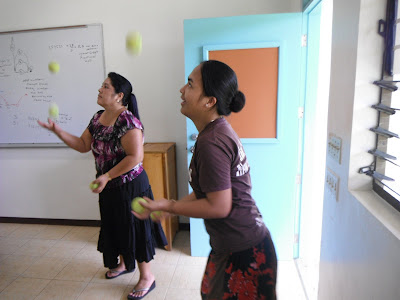Judy and May-vy

In an attempt to connect site swap theory back to the language of algebraic equations, after introducing site swap notation I referred to sequences such as 33342333 as site swap equations. The sequence is a mathematical statement that can be true (juggable) or false (not juggable).Arthy

This laboratory continues to provide a fun way to wrap up a term of exploring the mathematics at the core of physical science while expanding the students thinking with a mathematics system like nothing they have ever seen before.The laboratory is also an end of term enjoyable experience. As the course is not required by any major, the students are primarily from majors other than those in the natural sciences. For many of these students science is a requirement, possibly even a dreaded requirement.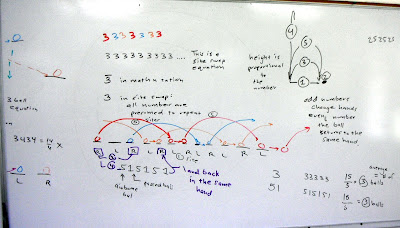I want the students to have the chance to do science, engage in exploring systems, grapple with the mathematical language underneath physical science. I can only hope that the students catch a glimpse of the beauty of science - of even pure science for science's sake.

1.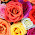Mathesmatics
Science and mathematics are not cool subjects, say students. Consequently, if these subjects are

compulsory, students opt for an easier stream in secondary school and are less likely to transition to

university science programs.

2.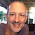While I do not set out to necessarily make science "cool," I do try to include some fun in the science with a laboratory such as the site swap laboratory.